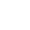﻿

/    2019-02-28

`package mainimport (    "fmt")type T struct {    string}func (t T) testT() {    fmt.Println("接受者为 T ")}func (t *T) testP() {    fmt.Println("接受者为 *T ")}func main() {    t := T{"oldboy"}    expression1 := T.testT    expression1(t)    expression2 := (*T).testT    expression2(&t)    // expression3 := T.testP    // expression3(t)    expression4 := (*T).testP    expression4(&t)}`

`package mainimport (    "fmt")type ST struct {    T}type SP struct {    *T}type T struct {    string}func (t T) testT() {    fmt.Println("类型 S 包含匿名字段 *T 或 T ，则 S 和 *S 方法集包含 T 方法")}func (t *T) testP() {    fmt.Println("类型 S 包含匿名字段 *T 或 T ，则 S 和 *S 方法集包含 *T 方法")}func main() {    st1 := ST{T{"oldboy"}}    expression1 := ST.testT    expression1(st1)    expression2 := (*ST).testT    expression2(&st1)    // expression3 := ST.testP    // expression3(st1)    expression4 := (*ST).testP    expression4(&st1)    sp1 := SP{&T{"oldboy"}}    expression5 := SP.testT    expression5(sp1)    expression6 := (*SP).testT    expression6(&sp1)    expression7 := SP.testP    expression7(sp1)    expression8 := (*SP).testP    expression8(&sp1)}`(2)

﻿